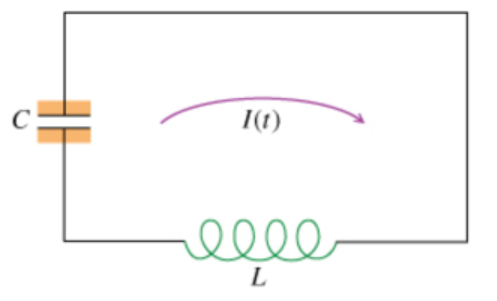# Problem: Consider an L-C circuit with capacitance C, inductance L, and no voltage source, as shown in the figure (Figure 1). As a function of time, the charge on the capacitor is Q(t) and the current through the inductor is I(t). Assume that the circuit has no resistance and that at one time the capacitor was charged.Part A. As a function of time, what is the energy UL(t) stored in the inductor? Express your answer in terms of L and I(t).UL(t) = Part B. As a function of time, what is the energy UC(t) stored in the inductor? Express your answer in terms of C and Q(t).UC(t) =

###### FREE Expert Solution

Energy stored in a magnetic field:

$\overline{){\mathbf{U}}{\mathbf{=}}\frac{\mathbf{1}}{\mathbf{2}}{\mathbf{L}}{{\mathbf{i}}}^{{\mathbf{2}}}}$

Energy stored in a capacitor:

$\overline{){\mathbf{U}}{\mathbf{=}}\frac{\mathbf{Q}\mathbf{V}}{\mathbf{2}}{\mathbf{=}}\frac{\mathbf{C}{\mathbf{V}}^{\mathbf{2}}}{\mathbf{2}}{\mathbf{=}}\frac{{\mathbf{Q}}^{\mathbf{2}}}{\mathbf{2}\mathbf{C}}}$

93% (459 ratings)###### Problem Details

Consider an L-C circuit with capacitance C, inductance L, and no voltage source, as shown in the figure (Figure 1). As a function of time, the charge on the capacitor is Q(t) and the current through the inductor is I(t). Assume that the circuit has no resistance and that at one time the capacitor was charged.Part A. As a function of time, what is the energy UL(t) stored in the inductor?

Express your answer in terms of L and I(t).

UL(t) =

Part B. As a function of time, what is the energy UC(t) stored in the inductor? Express your answer in terms of C and Q(t).

UC(t) =

Frequently Asked Questions

What scientific concept do you need to know in order to solve this problem?

Our tutors have indicated that to solve this problem you will need to apply the LC Circuits concept. You can view video lessons to learn LC Circuits. Or if you need more LC Circuits practice, you can also practice LC Circuits practice problems.

What professor is this problem relevant for?

Based on our data, we think this problem is relevant for Professor Jimenez's class at USF.